Скачать презентацию Tuesday October 19 Fill in planner

e877f0dc91c48a73ebcfee0865f9592a.ppt

• Количество слайдов: 54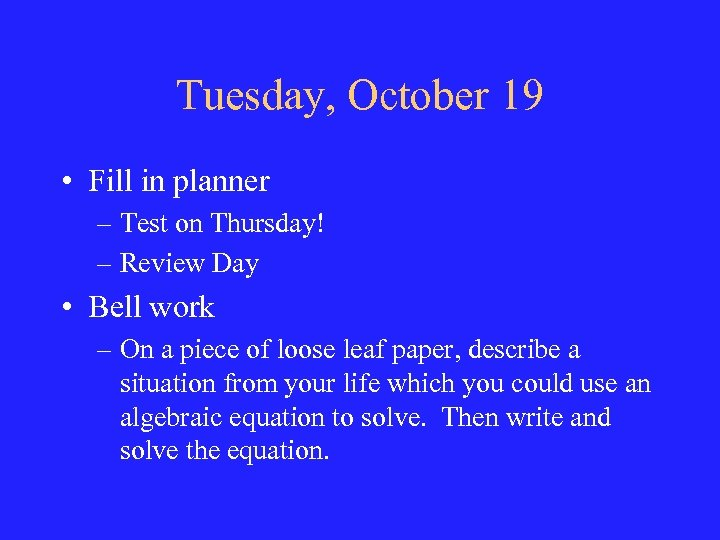Tuesday, October 19 • Fill in planner – Test on Thursday! – Review Day • Bell work – On a piece of loose leaf paper, describe a situation from your life which you could use an algebraic equation to solve. Then write and solve the equation.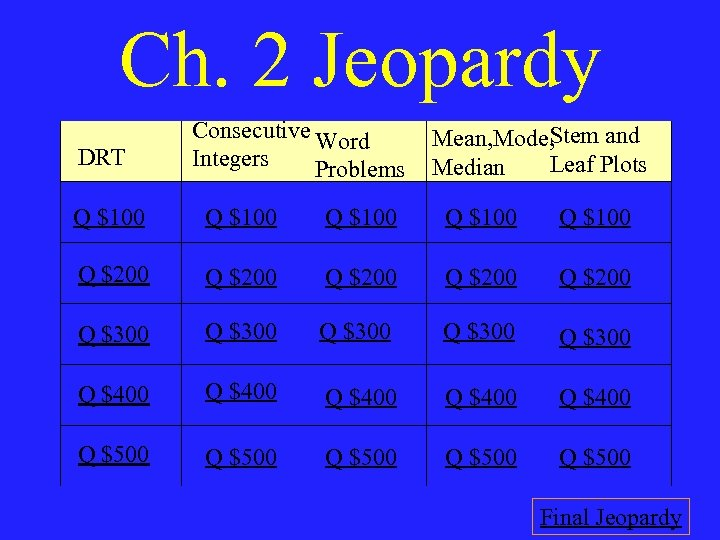Ch. 2 Jeopardy DRT Consecutive Word Integers Problems Mean, Mode, Stem and Leaf Plots Median Q \$100 Q \$100 Q \$200 Q \$200 Q \$300 Q \$300 Q \$400 Q \$400 Q \$500 Q \$500 Final Jeopardy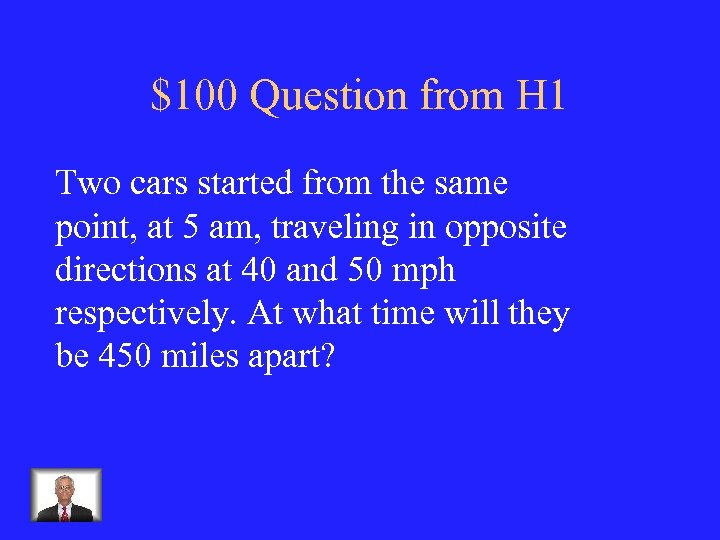\$100 Question from H 1 Two cars started from the same point, at 5 am, traveling in opposite directions at 40 and 50 mph respectively. At what time will they be 450 miles apart?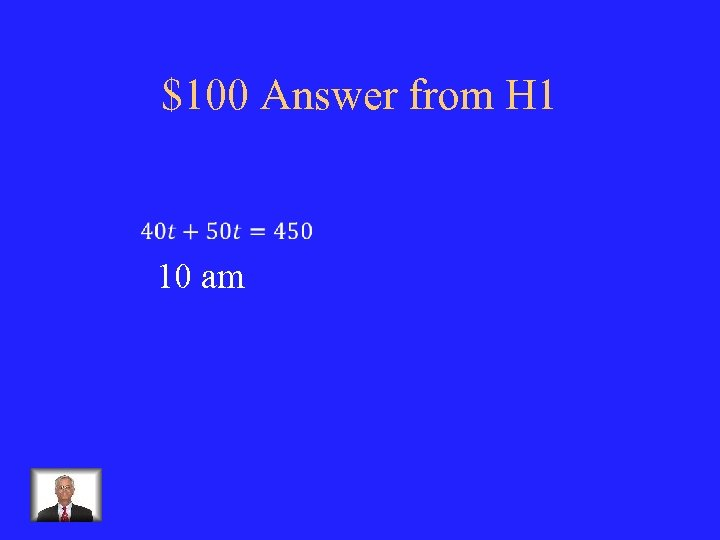\$100 Answer from H 1 10 am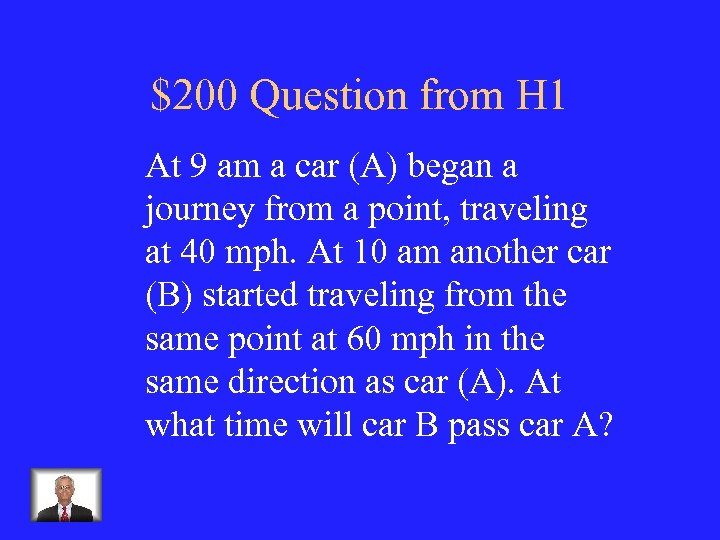\$200 Question from H 1 At 9 am a car (A) began a journey from a point, traveling at 40 mph. At 10 am another car (B) started traveling from the same point at 60 mph in the same direction as car (A). At what time will car B pass car A?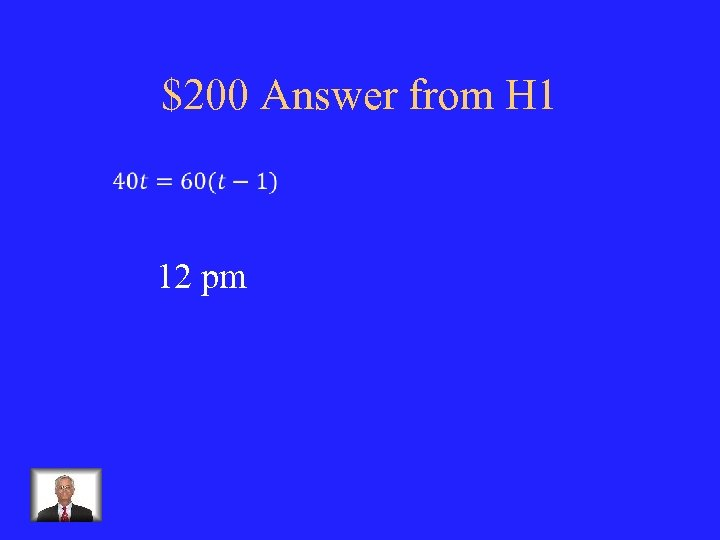\$200 Answer from H 1 12 pm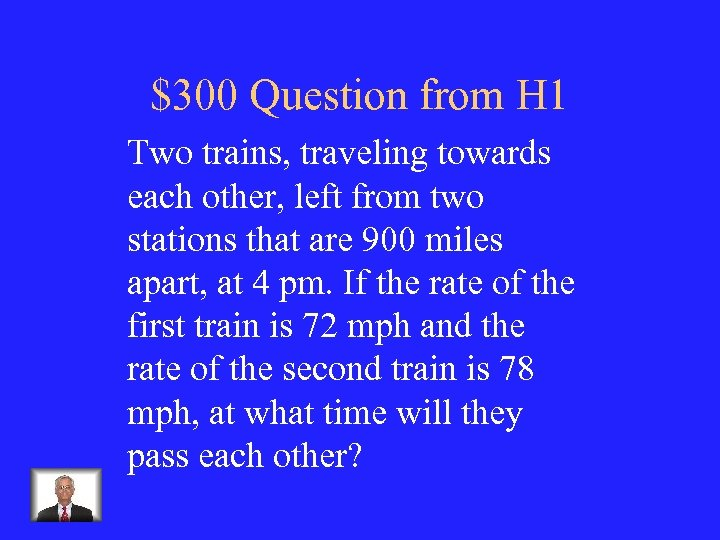\$300 Question from H 1 Two trains, traveling towards each other, left from two stations that are 900 miles apart, at 4 pm. If the rate of the first train is 72 mph and the rate of the second train is 78 mph, at what time will they pass each other?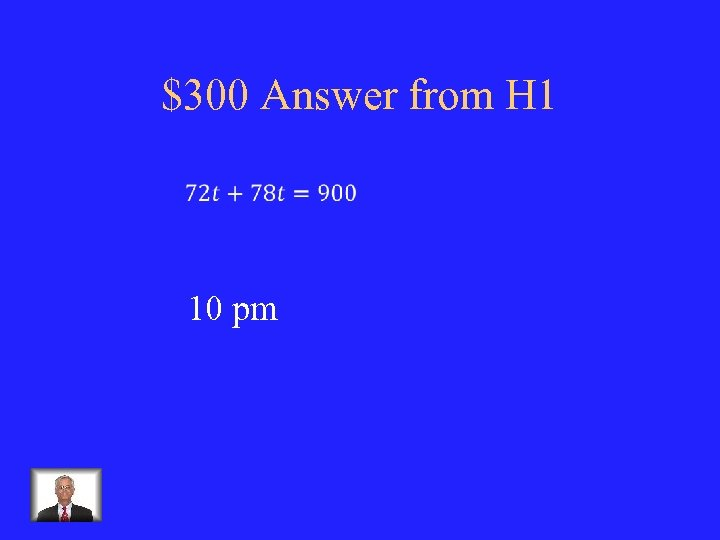\$300 Answer from H 1 10 pm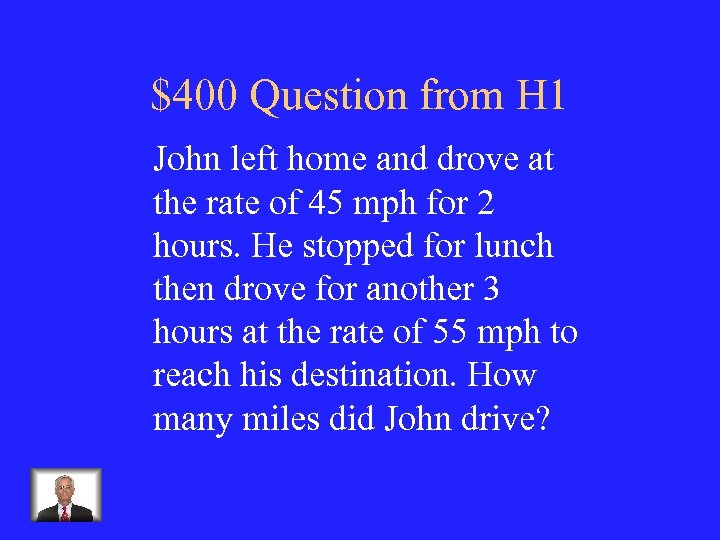\$400 Question from H 1 John left home and drove at the rate of 45 mph for 2 hours. He stopped for lunch then drove for another 3 hours at the rate of 55 mph to reach his destination. How many miles did John drive?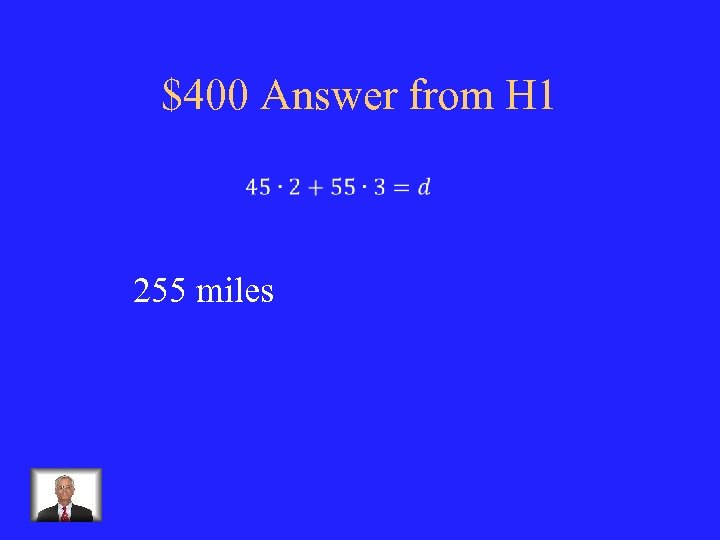\$400 Answer from H 1 255 miles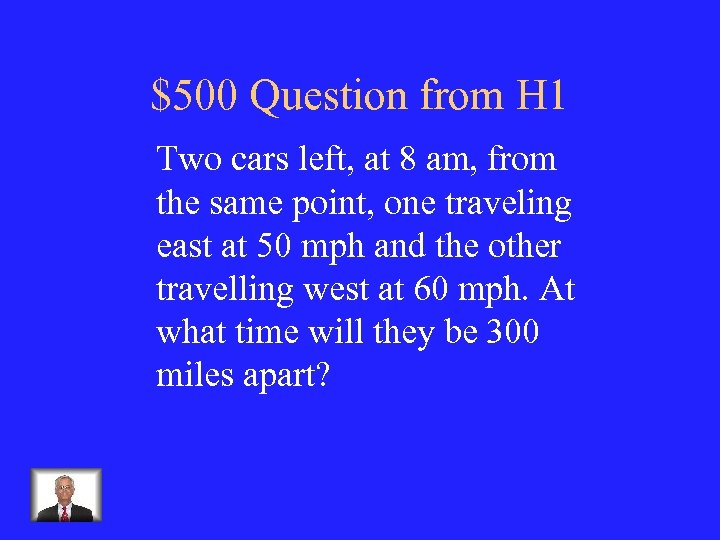\$500 Question from H 1 Two cars left, at 8 am, from the same point, one traveling east at 50 mph and the other travelling west at 60 mph. At what time will they be 300 miles apart?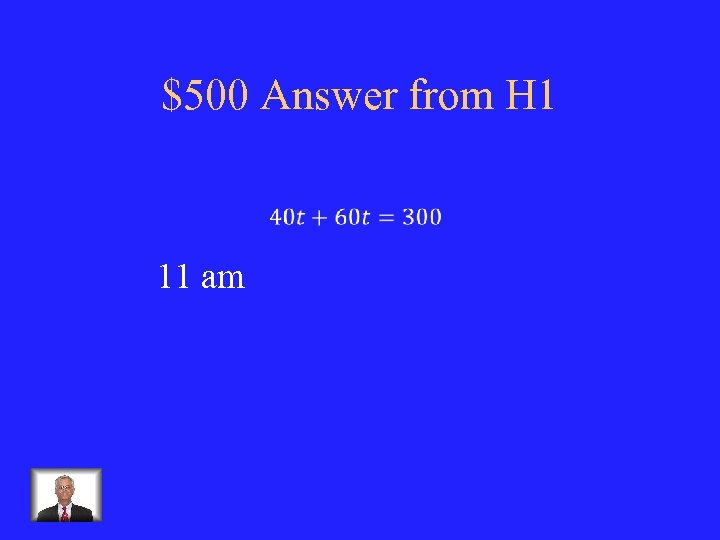\$500 Answer from H 1 11 am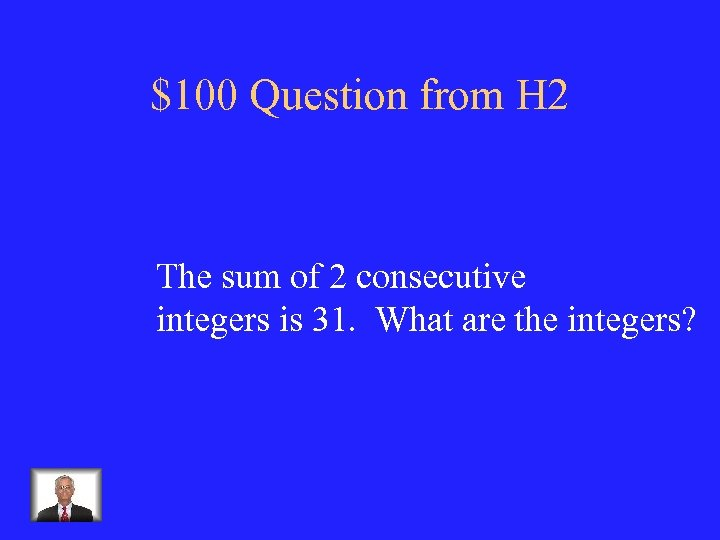\$100 Question from H 2 The sum of 2 consecutive integers is 31. What are the integers?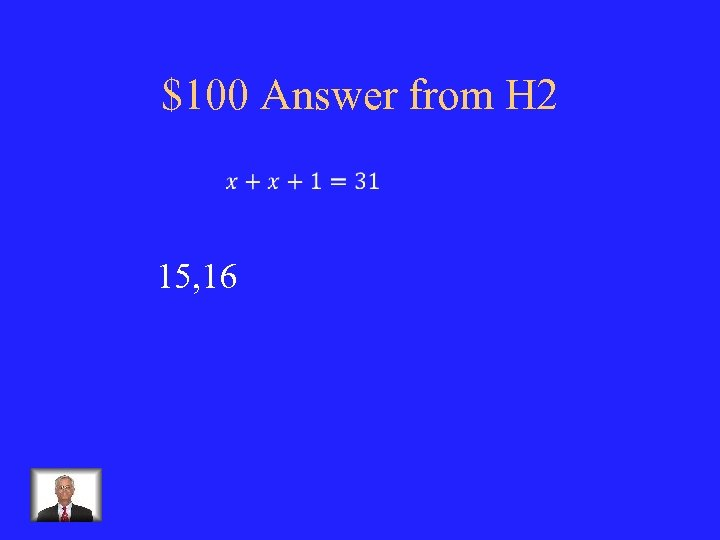\$100 Answer from H 2 15, 16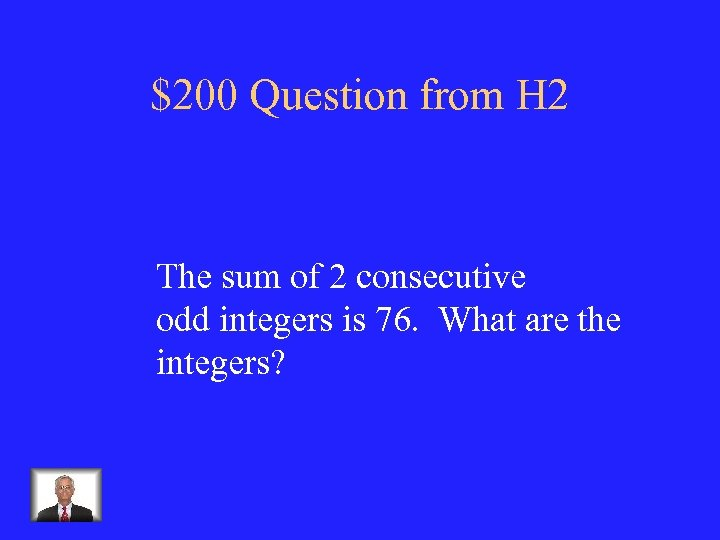\$200 Question from H 2 The sum of 2 consecutive odd integers is 76. What are the integers?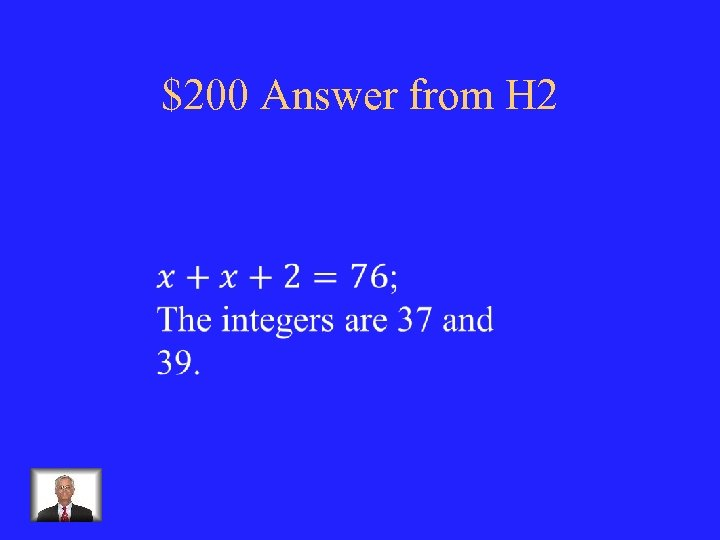\$200 Answer from H 2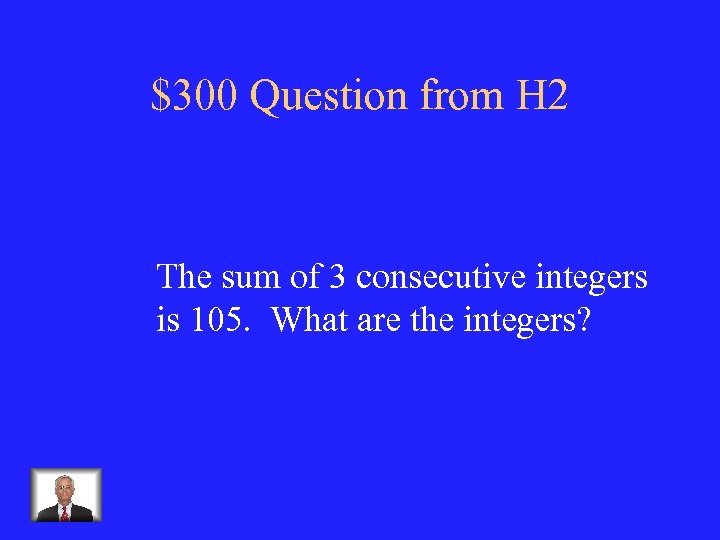\$300 Question from H 2 The sum of 3 consecutive integers is 105. What are the integers?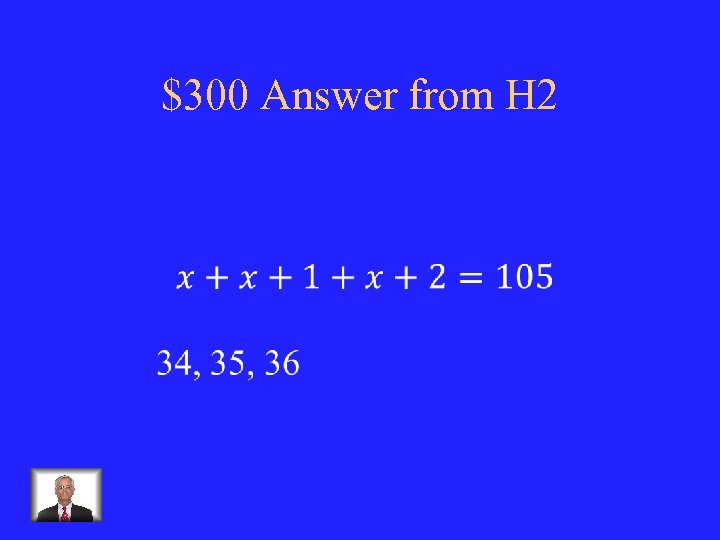\$300 Answer from H 2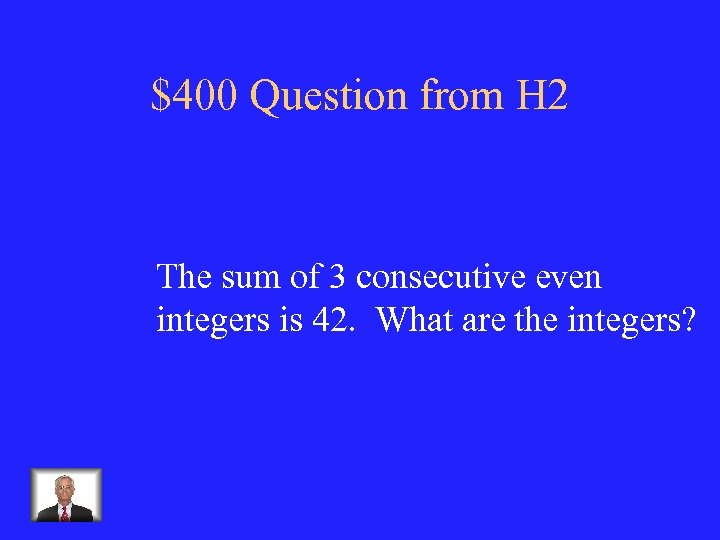\$400 Question from H 2 The sum of 3 consecutive even integers is 42. What are the integers?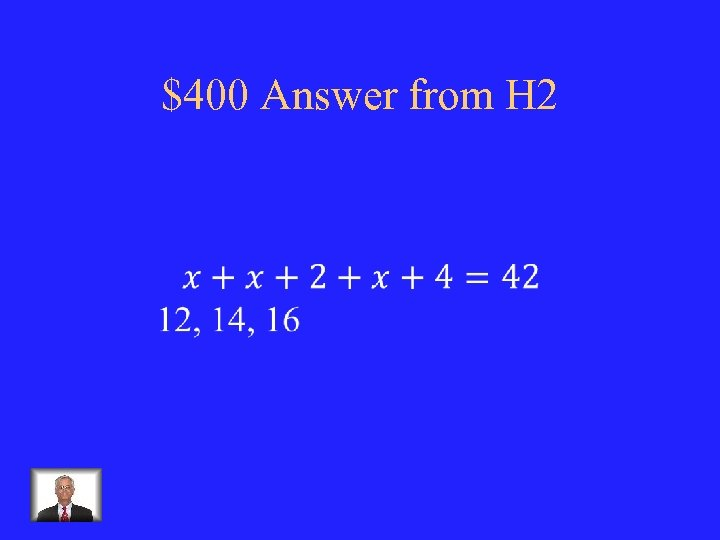\$400 Answer from H 2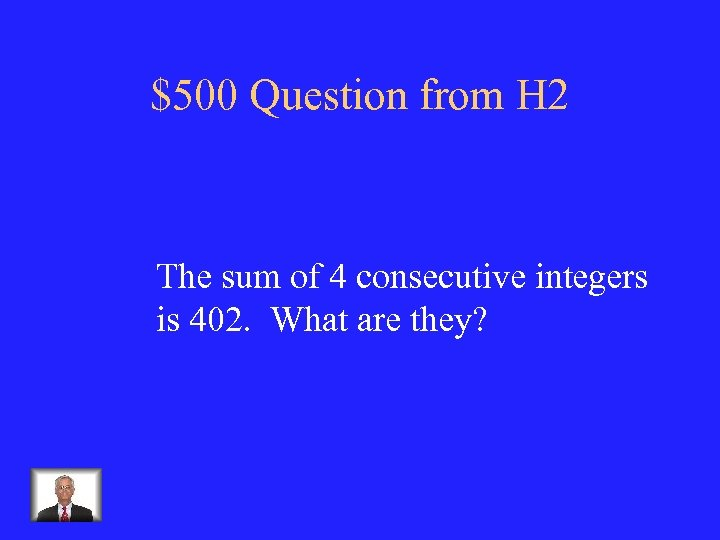\$500 Question from H 2 The sum of 4 consecutive integers is 402. What are they?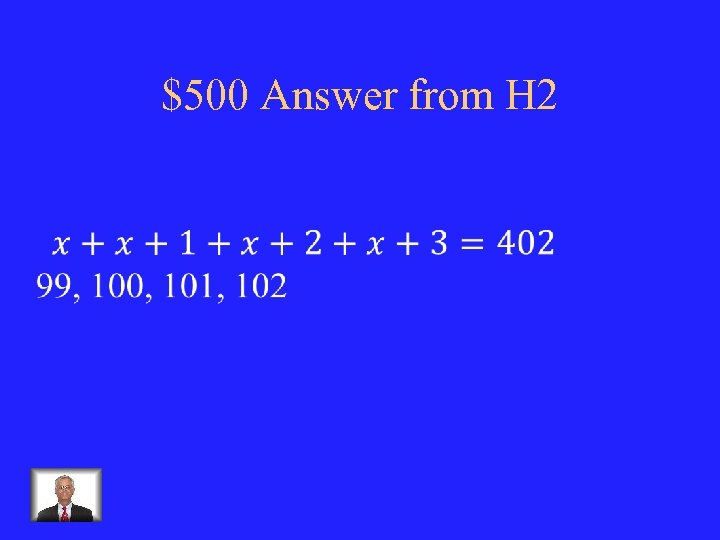\$500 Answer from H 2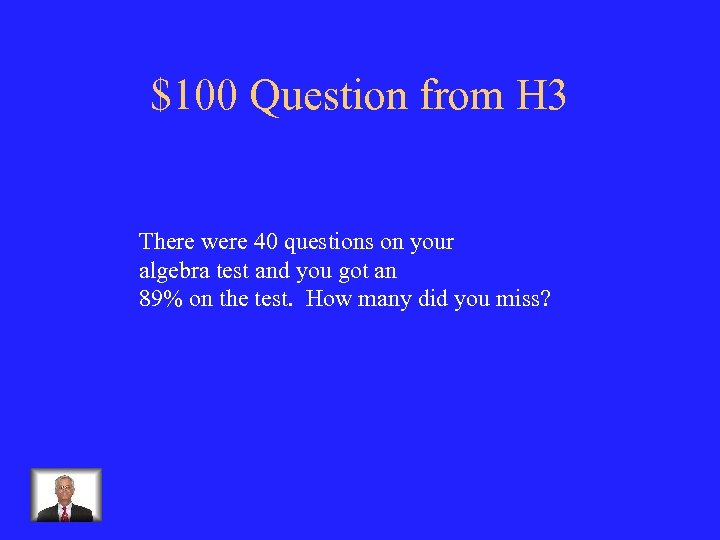\$100 Question from H 3 There were 40 questions on your algebra test and you got an 89% on the test. How many did you miss?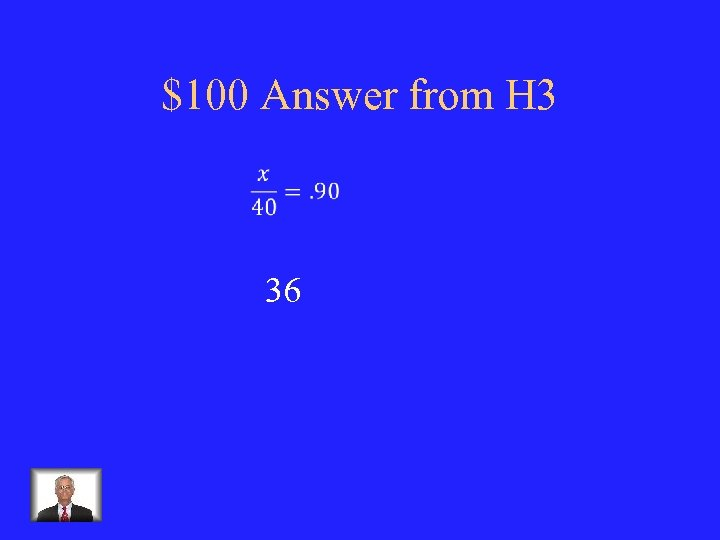\$100 Answer from H 3 36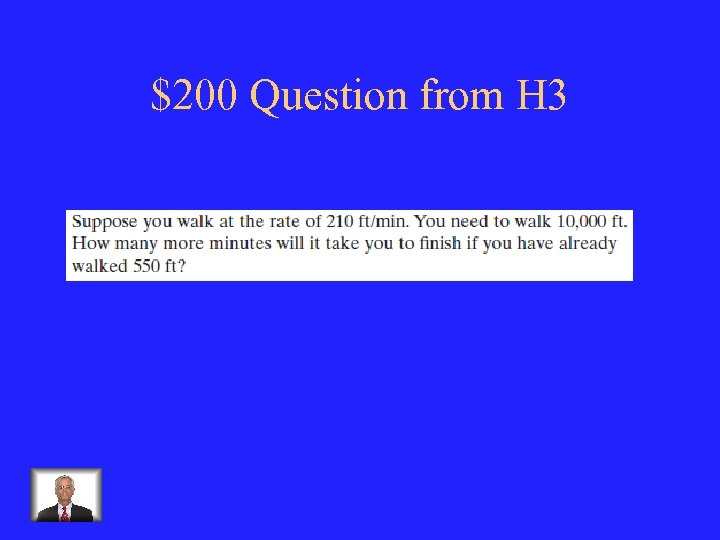\$200 Question from H 3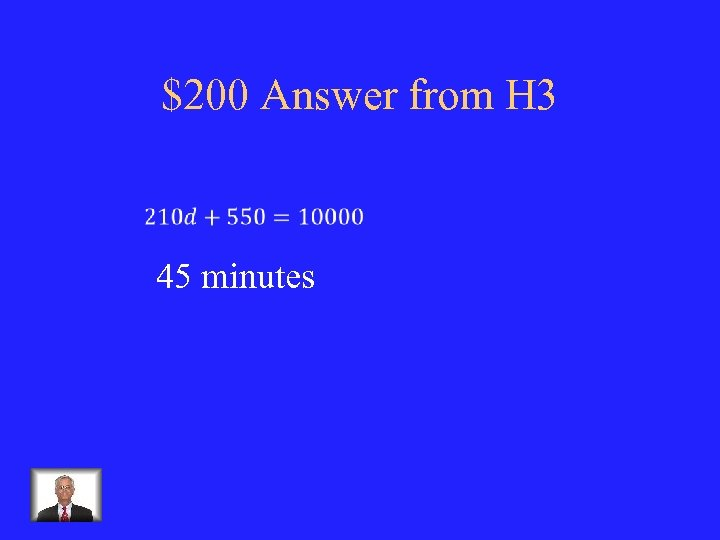\$200 Answer from H 3 45 minutes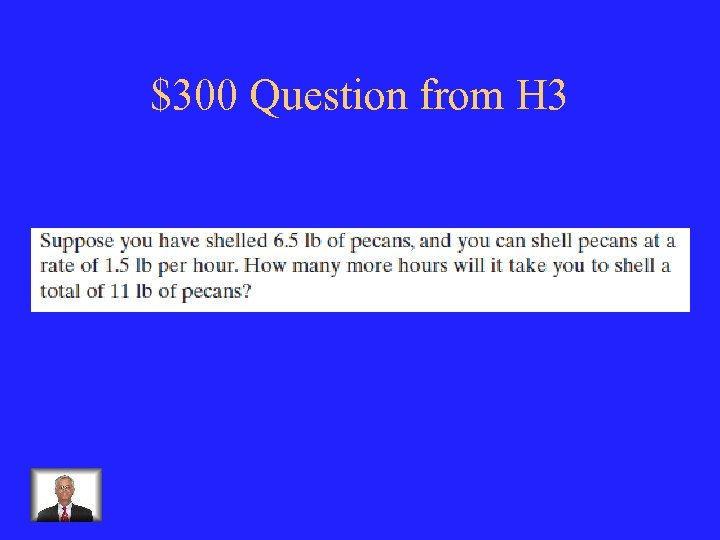\$300 Question from H 3 Your Text Here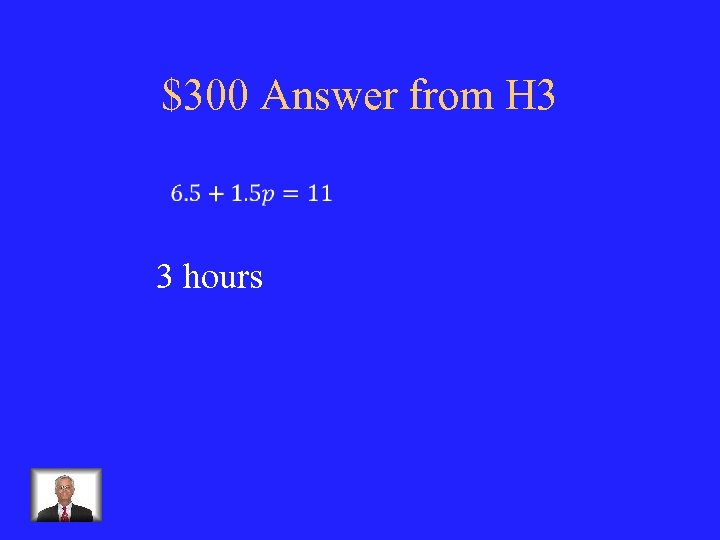\$300 Answer from H 3 3 hours\$400 Question from H 3 You can buy a skateboard from a friend for \$60 and rent safety equipment for \$1. 50/hr. Or you can rent all of your equipment for \$5. 50/hr. How many hours must you use your skateboard to justify buying your friend’s?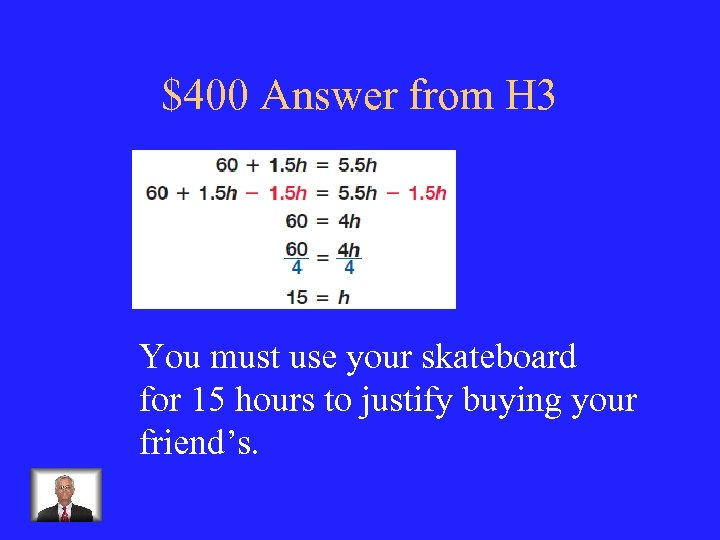\$400 Answer from H 3 You must use your skateboard for 15 hours to justify buying your friend’s.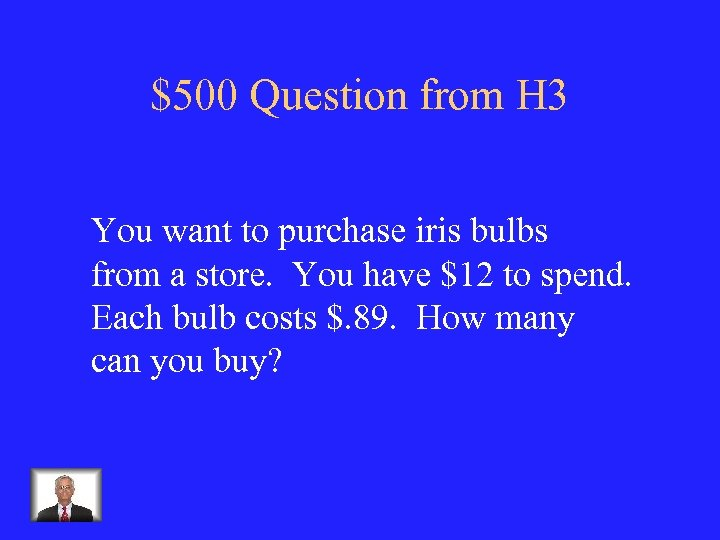\$500 Question from H 3 You want to purchase iris bulbs from a store. You have \$12 to spend. Each bulb costs \$. 89. How many can you buy?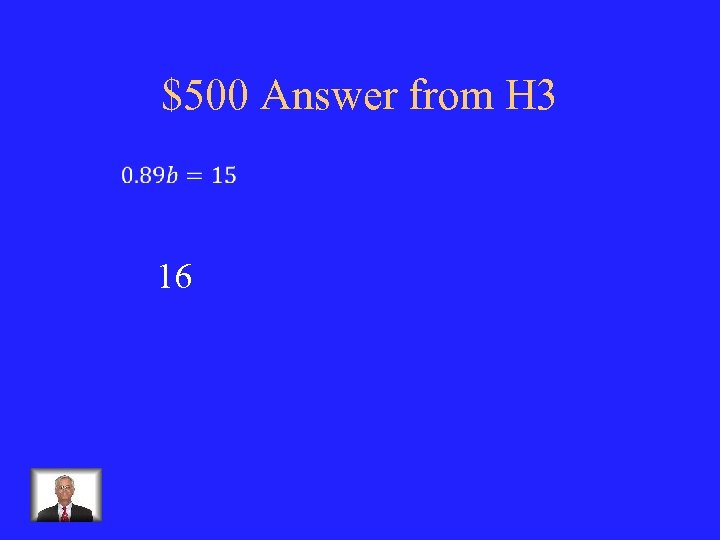\$500 Answer from H 3 16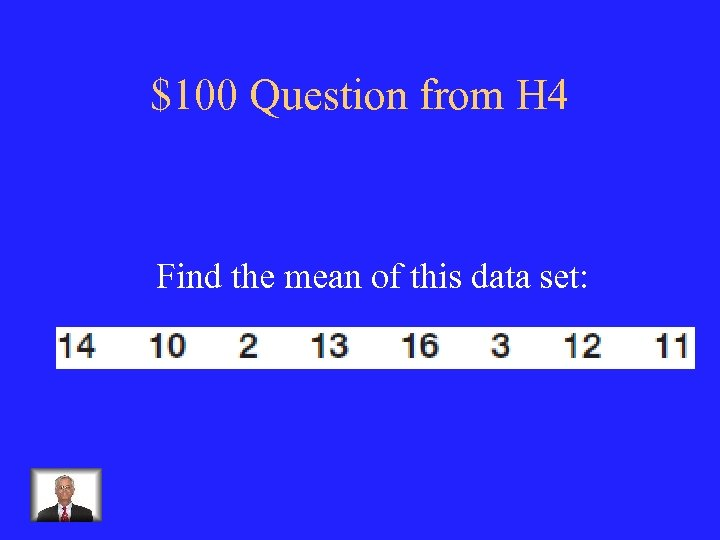\$100 Question from H 4 Find the mean of this data set: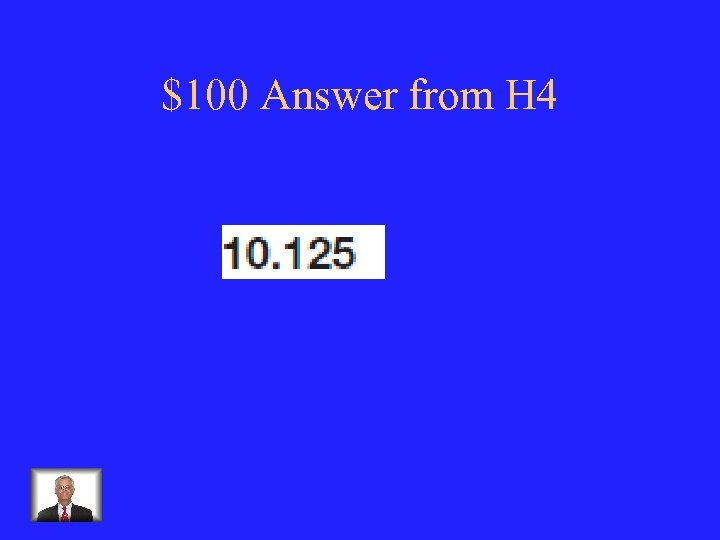\$100 Answer from H 4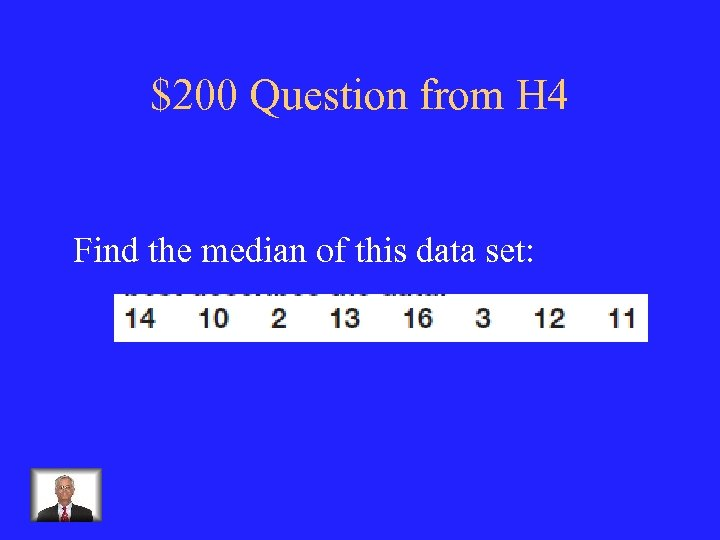\$200 Question from H 4 Find the median of this data set: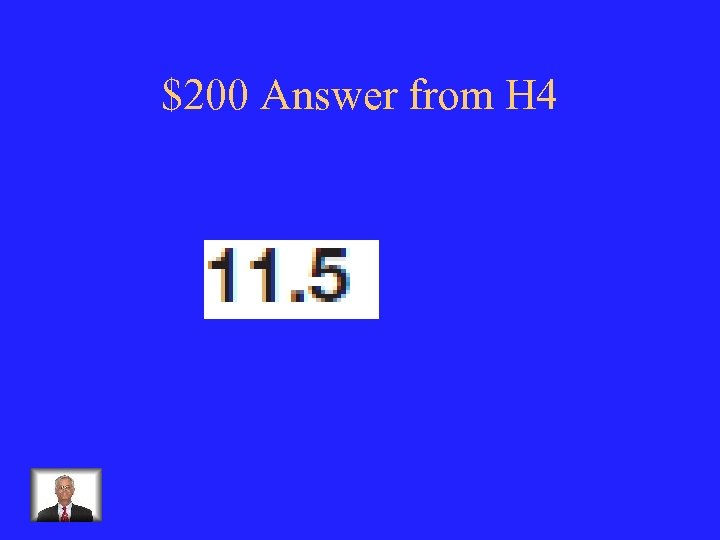\$200 Answer from H 4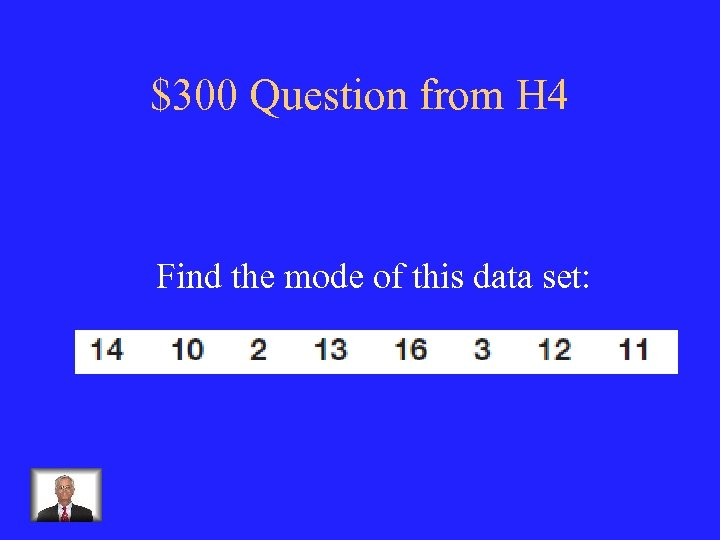\$300 Question from H 4 Find the mode of this data set: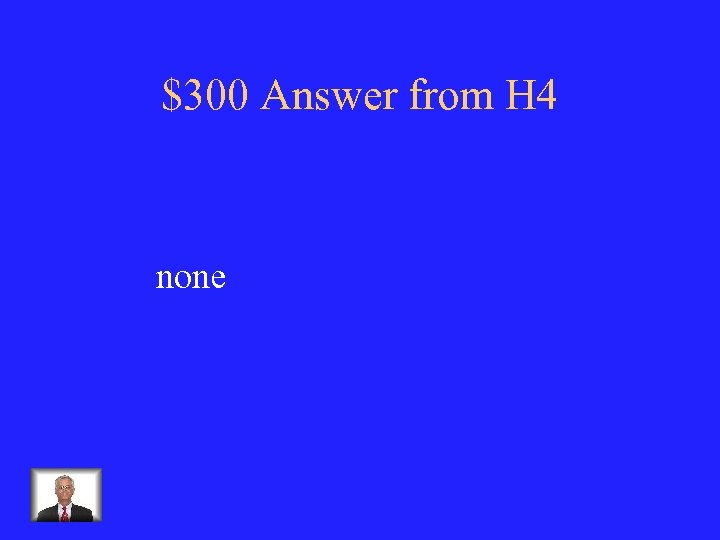\$300 Answer from H 4 none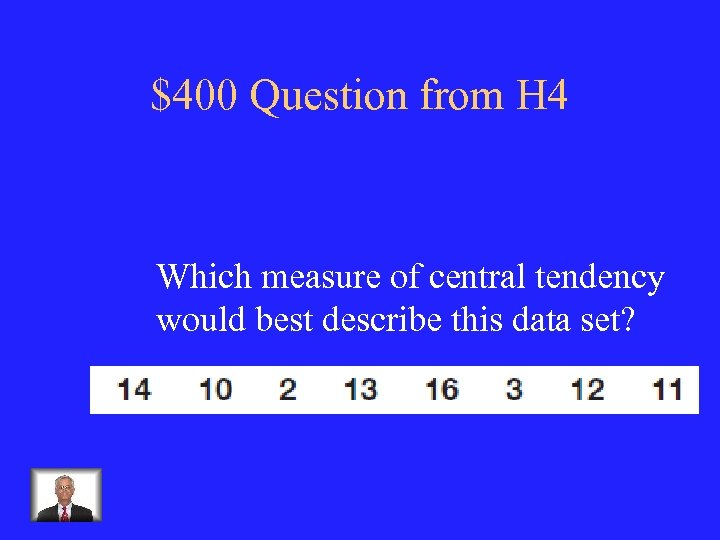\$400 Question from H 4 Which measure of central tendency would best describe this data set?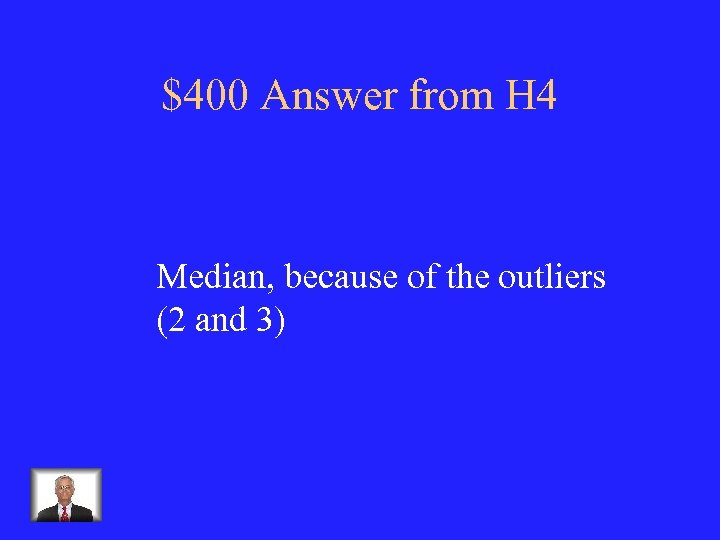\$400 Answer from H 4 Median, because of the outliers (2 and 3)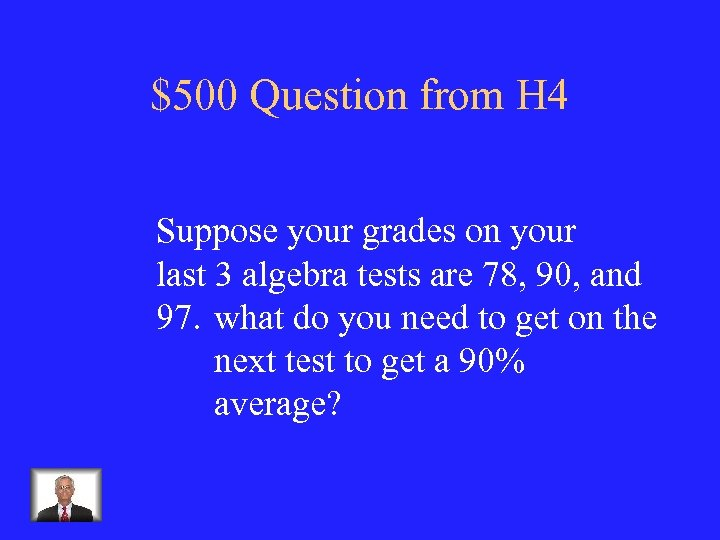\$500 Question from H 4 Suppose your grades on your last 3 algebra tests are 78, 90, and 97. what do you need to get on the next test to get a 90% average?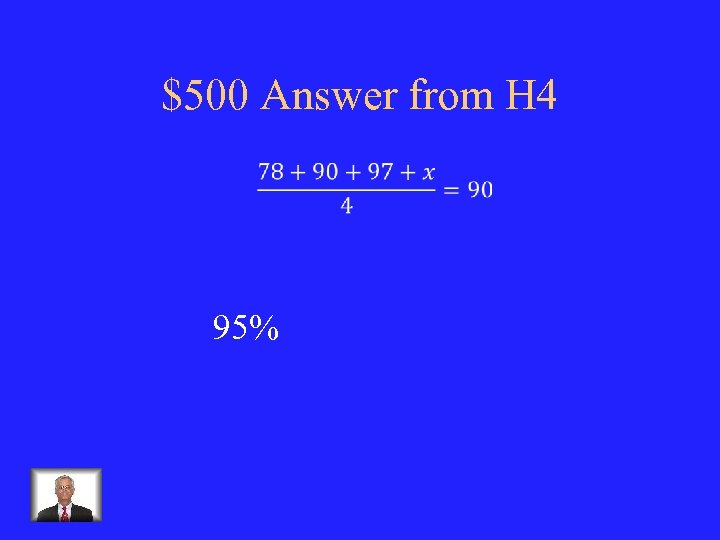\$500 Answer from H 4 95%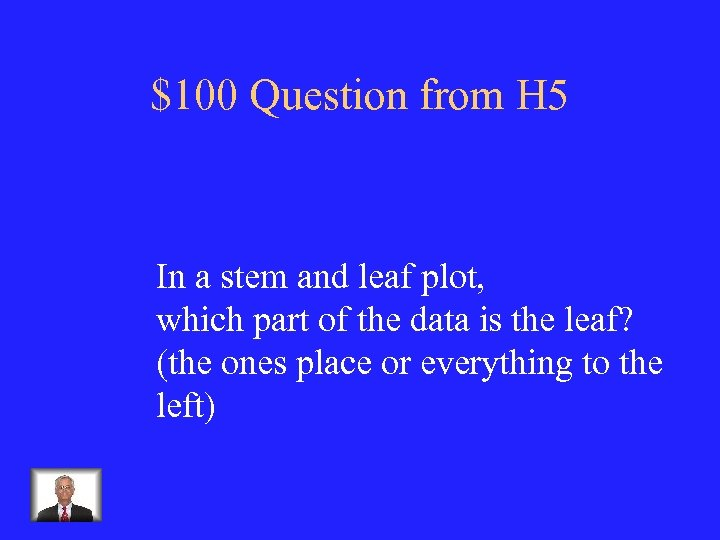\$100 Question from H 5 In a stem and leaf plot, which part of the data is the leaf? (the ones place or everything to the left)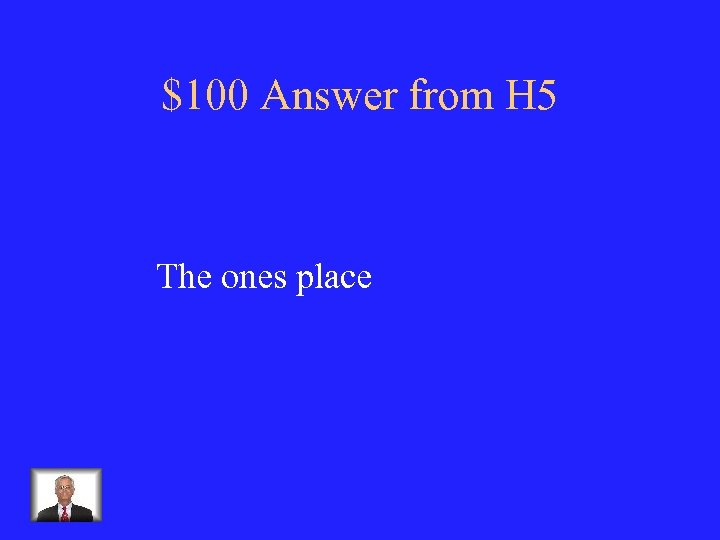\$100 Answer from H 5 The ones place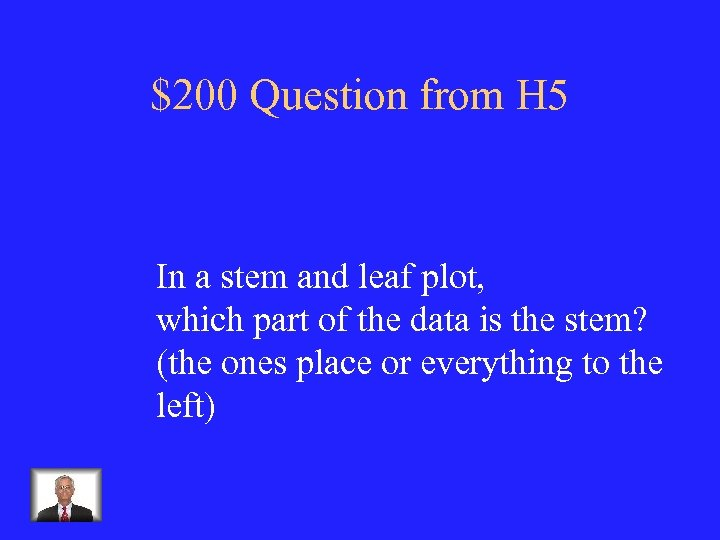\$200 Question from H 5 In a stem and leaf plot, which part of the data is the stem? (the ones place or everything to the left)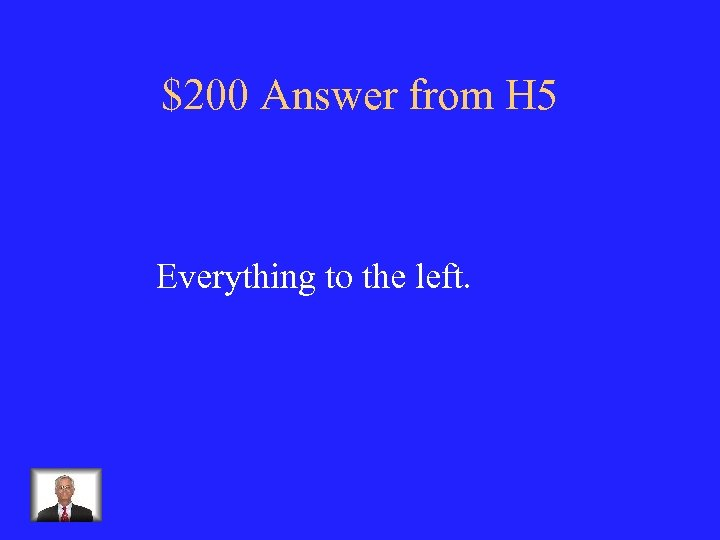\$200 Answer from H 5 Everything to the left.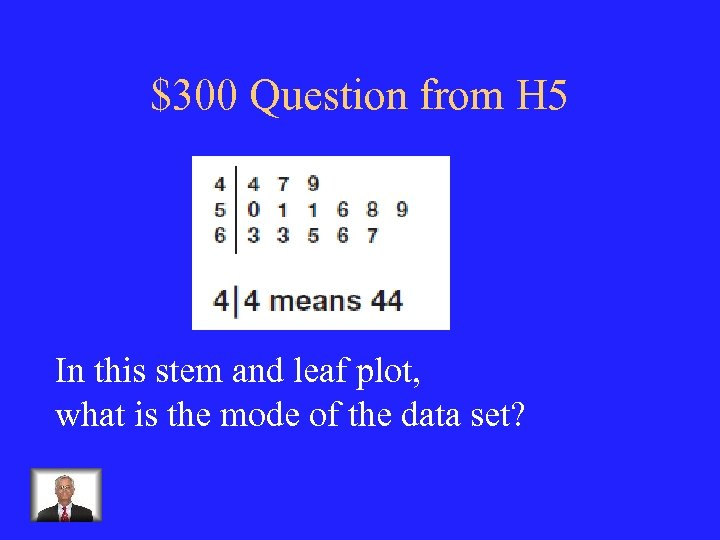\$300 Question from H 5 In this stem and leaf plot, what is the mode of the data set?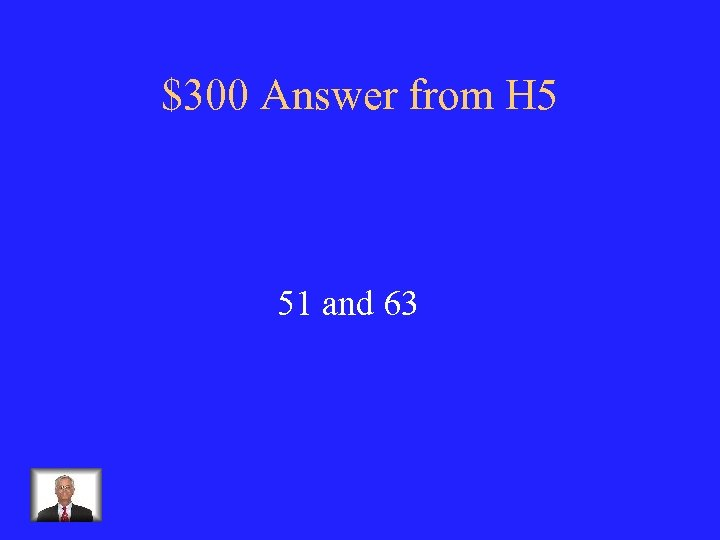\$300 Answer from H 5 51 and 63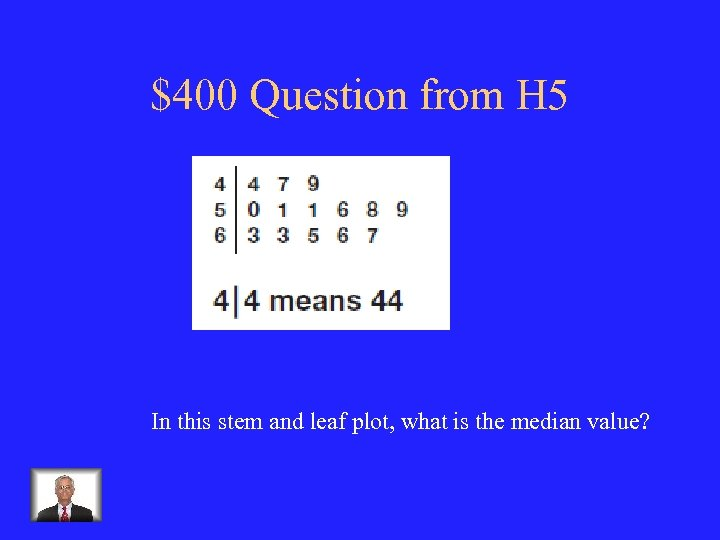\$400 Question from H 5 In this stem and leaf plot, what is the median value?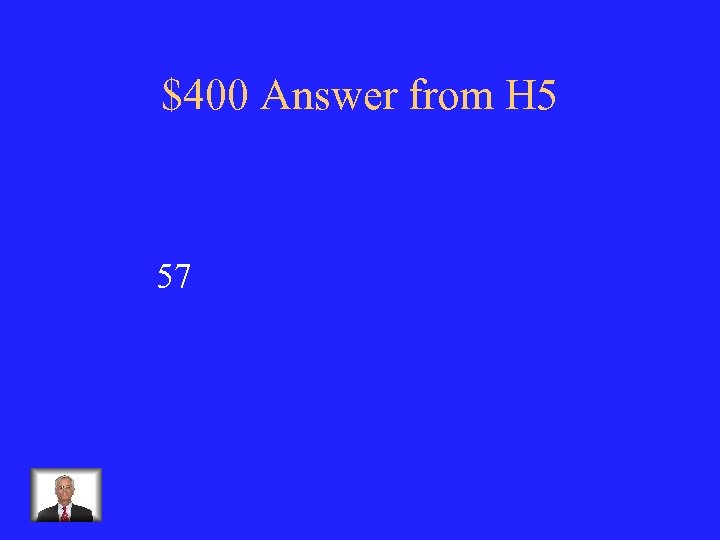\$400 Answer from H 5 57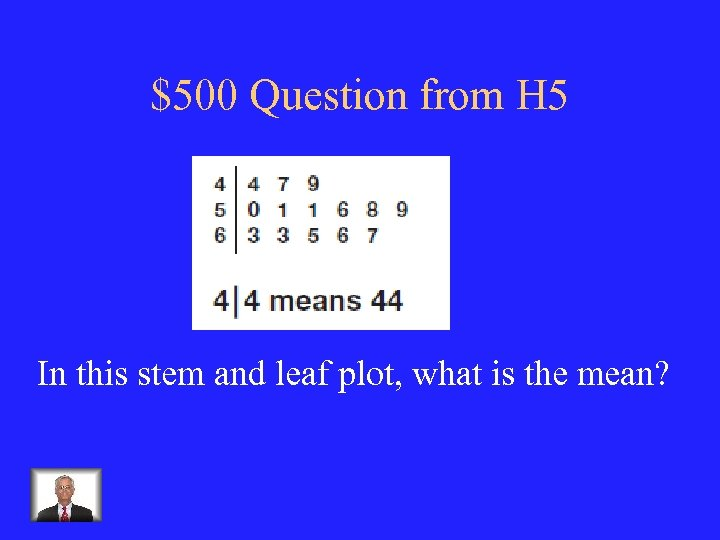\$500 Question from H 5 In this stem and leaf plot, what is the mean?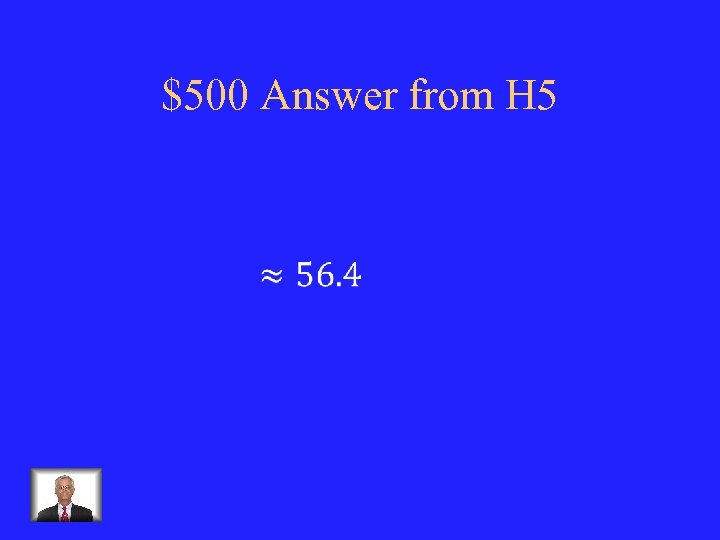\$500 Answer from H 5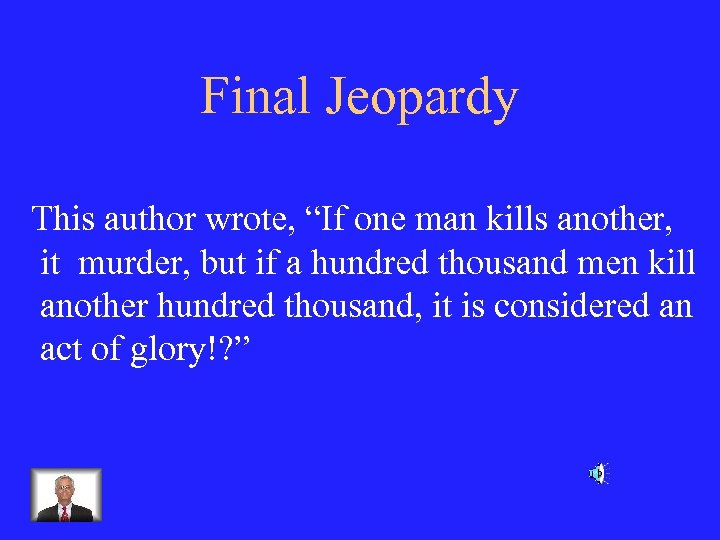Final Jeopardy This author wrote, “If one man kills another, it murder, but if a hundred thousand men kill another hundred thousand, it is considered an act of glory!? ”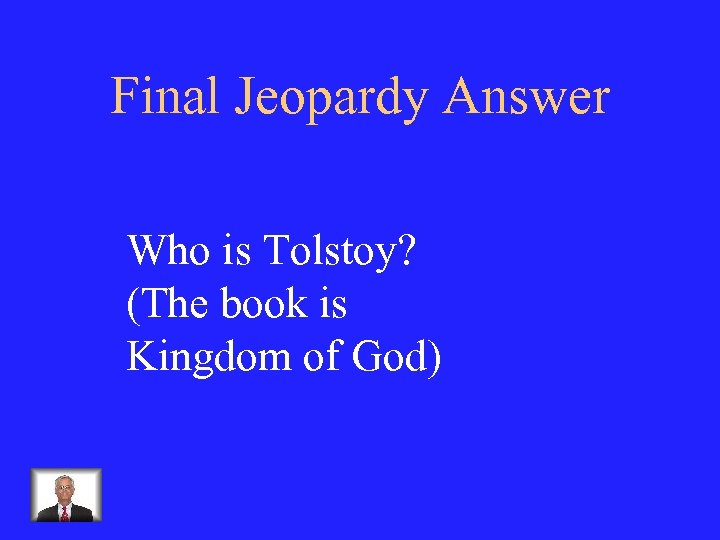Final Jeopardy Answer Who is Tolstoy? (The book is Kingdom of God)